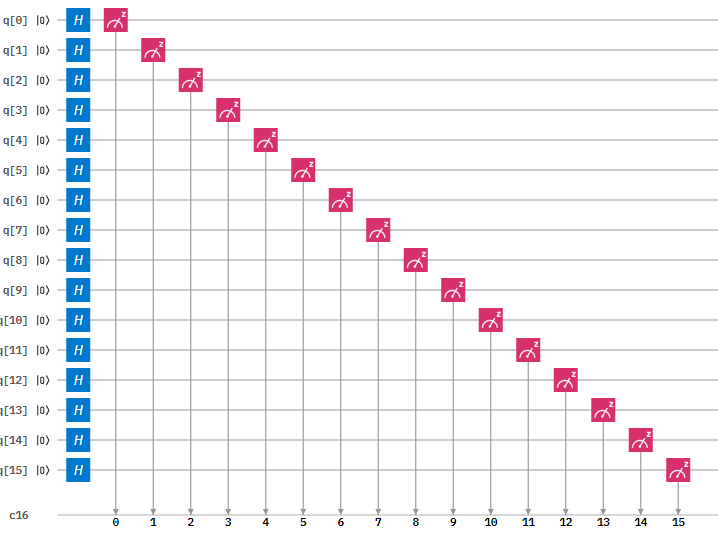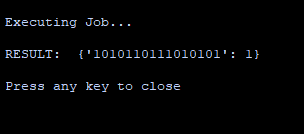# Quantum Programming 101: 16 Qubit Random Number Generator Tutorial# Installation

1. Install Python 3.x (Make sure Python is added to Path and Pip is checked)

2. Open Command Prompt and type in: pip install qiskit

3. Profit!

# ImplementationFigure 1: Circuit Diagram of the 16-qubit Random Number Generator

## STEP 1: INITIALISE THE QUANTUM AND CLASSICAL REGISTERS

The first step is to initialise a 16 qubit register . This is done by the following code:

q = QuantumRegister(16,’q’)

Next we initialise the 16 bit classical register with the following code:

c = ClassicalRegister(16,’c’)

## STEP 2: CREATE THE CIRCUIT

Next we create a quantum circuit using the following code:

circuit = QuantumCircuit(q,c)

## STEP 3: APPLY A HADAMARD GATE TO ALL QUBITS

Then we need to apply a Hadamard gate. This gate is used to put a qubit in to a superposition of 1 and 0 such that when we measure the qubit it will be 1 or a 0 with equal probability.

This is done with the following code:

circuit.h(q)

## STEP 4: MEASURE THE QUBITS

After this we measure the qubits. This measurement will collapse the qubits superposition in to either a 1 or a 0.

This is done with the following code:

circuit.measure(q,c)

# How to run the program

1. Copy and paste the code below in to a python file

2. Enter your API token in the IBMQ.enable_account(‘Insert API token here’) part

3. Save and run

# Code

``````from qiskit import QuantumRegister, ClassicalRegister, QuantumCircuit, execute,IBMQ

IBMQ.enable_account('ENTER API TOKEN HERE')
provider = IBMQ.get_provider(hub='ibm-q')

q = QuantumRegister(16,'q')
c = ClassicalRegister(16,'c')
circuit = QuantumCircuit(q,c)
circuit.h(q) # Applies hadamard gate to all qubits
circuit.measure(q,c) # Measures all qubits

backend = provider.get_backend('ibmq_qasm_simulator')
job = execute(circuit, backend, shots=1)

print('Executing Job...\n')
result = job.result()
counts = result.get_counts(circuit)

print('RESULT: ',counts,'\n')
print('Press any key to close')
input()``````

# Output

Once you have ran the program you will get the following output:Want to learn about Quantum Programming? Head over to Quantum Computing UK: https://quantumcomputinguk.org/#### Macauley Coggins

Macauley Coggins is a Software Developer, Researcher, and Managing Director of Quantum Computing UK. He has experience in developing software for IBMs Quantum Computers and has a special interest in developing secure cryptographic systems with quantum hardware.